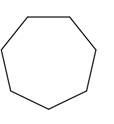Chapter 23, Problem 22PS

Chapter
Section
Textbook Problem

Draw a structure for cycloheptane. Is the seven-member ring planar? Explain your answer.

Interpretation Introduction

Interpretation:

The structure for cycloheptane has to be drawn. Cycloheptane is whether planar has to be explained briefly.

Concept introduction:

Cycloheptne is a cycloalkane. It is used as a non-polar solvent and molecular formula is C7H14.

It is a colourless oily liquid.

The structure of cycloheptane is as follows.In general, the cycloalkanes having more than six carbon atoms experience strain since the angles are too far from the preferred angle which is 109.5o so they are not planar.

Explanation

Cycloheptane is seven member ring and it is non planar. In cycloheptane all the carbon atoms are sp3 hybridized and there is tetrahedral geometry around each carbon. Therefore, it is non –planar.

The structure of cycloheptane is,

Still sussing out bartleby?

Check out a sample textbook solution.

See a sample solution

The Solution to Your Study Problems

Bartleby provides explanations to thousands of textbook problems written by our experts, many with advanced degrees!

Get Started

Find more solutions based on key concepts